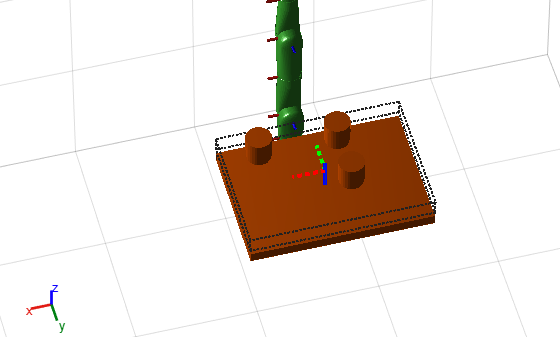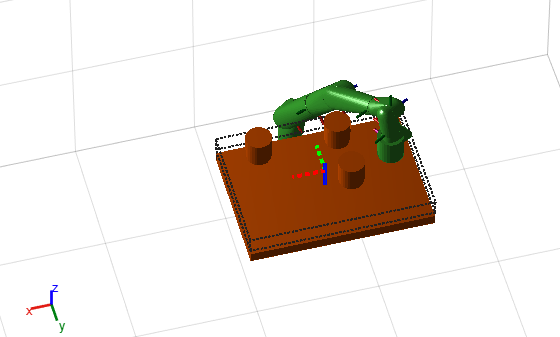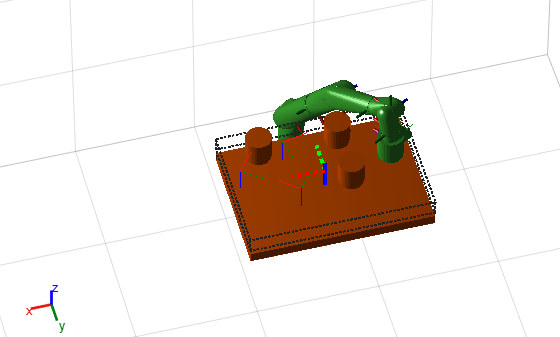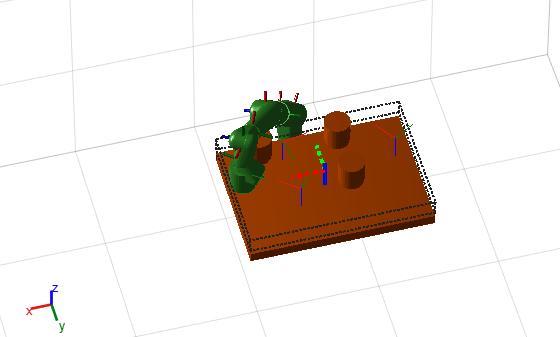# sample

Sample end-effector poses in world frame

Since R2021a

## Syntax

``eePose = sample(goalRegion)``
``eePose = sample(goalRegion,numSamples)``

## Description

````eePose = sample(goalRegion)` samples an end-effector pose in the world frame as a homogeneous transformation matrix. The function returns a pose uniformly sampled within the Bounds property relative to the reference frame and applies the following transformations based on the ReferencePose and EndEffectorOffsetPose properties: tSample; % Pose sampled within Bounds Tw0 = goalRegion.ReferencePose; TeW = goalRegion.EndEffectorOffsetPose; eePose = Tw0 * tSample * TeW; % tSample is a pose within the bounds. ```

example

````eePose = sample(goalRegion,numSamples)` samples multiple poses based on the input `numSamples`. The function returns the end-effector poses as a 3-D array of homogeneous transforms.```

## Examples

collapse all

Sample various poses within the bounds of a workspace goal region for a manipulator arm. Some end-effector poses may not be desirable due to the positioning of the arm bodies and obstacles in the scene. The `workspaceGoalRegion` object defines the bounds on the xyz-position and zyx Euler orientation of the robot end effector. The `sample` object function uniformly samples random poses within the bounds. Find configurations that achieve these end-effector poses and determine the best by visualization.

Load an existing robot model as a `rigidBodyTree` object.

```robot = loadrobot("kinovaGen3","DataFormat","row"); show(robot,"Collisions","on","Visuals","off");```

Add a can as a `collisionCylinder` object to the robot arm.

```can = collisionCylinder(0.05, 0.1); can.Pose = trvec2tform([0.2, 0.3, 0.5]); addCollision(robot.Bodies{end},"cylinder", [0.05, 0.1], trvec2tform([0, 0, 0.02])); ```

The goal of this example is to place this can on a table with other cans. Add the table and other cans to the environment by creating a cell array of collision objects. Show the entire `env` cell array.

```table = collisionBox(0.7, 0.5, 0.04); table.Pose = trvec2tform([0, 0.5, 0.43]); env = {can, copy(can), copy(can), table}; env{2}.Pose = trvec2tform([-0.1, 0.3, 0.5]); env{3}.Pose = trvec2tform([-0.1, 0.5, 0.5]); hold on for i = 1: length(env) show(env{i}) end show(robot,homeConfiguration(robot),"Collisions","on","Visuals","off"); ```Define Goal Region

Create a workspace goal region using the end-effector body name of the robot.

Define the goal region parameters for your workspace. The goal region includes a reference pose, xyz-position bounds, and orientation limits on the zyx Euler angles. This example specifies xyz bounds within the table dimensions and fixes rotation to a small range in the y- and x-axis.

```tableRegion = workspaceGoalRegion("EndEffector_Link",... "ReferencePose",table.Pose); tableRegion.EndEffectorOffsetPose(1:3,1:3) = eul2rotm([0, 0, pi]); tableRegion.EndEffectorOffsetPose(3, end) = 0.1; tableRegion.Bounds = ... [-table.X/2, table.X/2; % X Bounds -table.Y/2, table.Y/2; % Y Bounds 0.04, 0.10; % Z Bounds -pi, pi; % Rotation about Z-axis -0.01, 0.01; % Y-Axis -0.01, 0.01;]; % X-Axis show(tableRegion); view(165,50) camzoom(3.5)```Sample Poses

Uniformly sample poses within the table region using the `sample` object function. In this example, set the `rng` seed to get repeatable results. Create vectors for storing valid and invalid poses.

```rng(0) poses = sample(tableRegion,10); validPoses = []; invalidPoses = [];```

Check for Collisions

To find configurations for those poses, create an inverse kinematics (IK) solver.

```ik = inverseKinematics('RigidBodyTree',robot); config = cell(10);```

Test the sampled poses by iterating through the sampled poses, solving for configurations using IK, and checking for collisions. Show the valid configurations.

```for i = 1:length(poses) % Solve for robot configuraiton using IK. config{i} = ik("EndEffector_Link",poses(:,:,i),ones(6,1),homeConfiguration(robot)); % Check for collisions. isColliding = checkCollision(robot,config{i},env,SkippedSelfCollisions="parent"); if ~isColliding % If not in collision, show robot configuration and save valid pose. show(robot,config{i},"PreservePlot",false,"Collisions","on","Visuals","off"); drawnow validPoses = [validPoses; i]; else invalidPoses = [invalidPoses; i]; end end````disp(string(validPoses'))`
``` "3" "5" "7" "10" ```

Visualize A Singe Valid Pose

Plot all valid poses as transforms. The final valid configuration from checking collisions is still visible in the figure.

```translations = tform2trvec(poses(:,:,validPoses)); rotations = tform2quat(poses(:,:,validPoses)); plotTransforms(translations,rotations,"FrameSize",0.1)```Show a valid configuration from the list. Change the index in `validPoses` to look at different poses. Call `hold off` to stop preserving figure elements. To manually inspect poses and configurations, comment out the final line when running.

```poseIndex = validPoses(1); show(robot,config{poseIndex},"PreservePlot",false,"Collisions","on","Visuals","off"); hold off```## Input Arguments

collapse all

Workspace goal region, specified as a `workspaceGoalRegion` object.

Number of samples, specified as a positive integer

## Output Arguments

collapse all

Poses sampled within the workspace bounds in the world frame, returned as a four-by-four homogeneous transformation matrix or 4-by-4-by-n array, where n is the number of samples `numSamples`.

The function returns a pose uniformly sampled within the Bounds property relative to the reference frame and applies the following transformations based on the ReferencePose and EndEffectorOffsetPose properties:

```tSample = rand(6,2); Tw0 = goalRegion.ReferencePose; TeW = goalRegion.EndEffectorOffsetPose; eePose = Tw0 * tSample * TeW;```

Data Types: `double`

## Version History

Introduced in R2021a